# Thermodynamic Phases

• Rahulx084

#### Rahulx084

#Can somebody please explain what is the difference between single phase and homogeneous phase in context with thermodynamics?
#Also in the fundamental relations in thermodynamics like dg=vdp-sdT , it says this is applicable to homogeneous phase of constant composition.
Isn't this equation applicable to single component only cause in multi component we have chemical potential sort of thing in fundamental relations then what does the term constant composition means for a single component system?
Also it further talks about applicability pf this relation to close system or open system with constant composition.

Please make me understand these terminologies using some examples, that would be great.

#Can somebody please explain what is the difference between single phase and homogeneous phase in context with thermodynamics?
Single phase means that the material is all solid, liquid, or gas. Homogeneous means that the material is spatially uniform.
#Also in the fundamental relations in thermodynamics like dg=vdp-sdT , it says this is applicable to homogeneous phase of constant composition.
Isn't this equation applicable to single component only cause in multi component we have chemical potential sort of thing in fundamental relations then what does the term constant composition means for a single component system?
Constant composition means that the mole fractions of all species in the mixture do not change. If that is the case, the chemical potential terms do not contribute to the free energy per mole of mixture. For a single component, its mole fraction is always 1.
Also it further talks about applicability pf this relation to close system or open system with constant composition.
For an open system with constant mole fractions of the species, the free energy per mole of mixture depends only on temperature and pressure, and not on the number of moles of the mixture.

Can we say liq-vap water system is a homogeneous phase with constant composition system?

Can we say liq-vap water system is a homogeneous phase with constant composition system?
It is 2-phase, and each phase separately is homogeneous.

It is 2-phase, and each phase separately is homogeneous.

Then how we do use this equation during a phase change operation and say as temperature and pressure is constant ,gibbs free energy change is 0.
Here is what I'm making out , that we apply dg(vapour) separately and dg(liquid) separately and for total change we do , dg= dg(vap) +dg(liq) , and this is equal to 0. Is it like this ? That we can't apply dg for the whole system cause its two different phases? Or we can considering the whole system is closed but then what about the condition of homogeneous phase and constant composition ? Can we as a whole consider it as homogeneous phase?

For a phase change, we do the phase change at constant temperature and pressure, and, when done reversibly, using the 1st and 2nd laws of thermodynamics, we get:
$$q=\Delta H=H_V-H_L$$
$$\Delta S=S_V-S_L=\frac{q}{T}=\frac{\Delta H}{T}$$
$$\Delta G = G_V-G_L=\Delta H-T\Delta S=0$$
where ##H_V## and ##H_L## represent the enthalpy of one mole of vapor (the final state) and the enthalpy of one mole of liquid (the initial state), respectively, at temperature T and P. Similar definition apply to the initial and final states for entropy change and Gibbs free energy change at T and P.

So where does dG=-SdT+VdP come in? Suppose we wanted to determine how the equilibrium vapor pressure changes with temperature T along the saturation line. For each of the two homogeneous phases separately, we could write: $$dG_V=-S_VdT+V_VdP\tag{1}$$and$$dG_L=-S_LdT+V_LdP\tag {2}$$ where ##V_V## and ##V_L## are the molar volumes of the saturated vapor and saturated liquid, respectively. Then, if we subtract Eqn. 2 from Eqn. 1, we obtain:
$$dG_V-dG_L=d(G_V-G_L)=d(\Delta G)=-\Delta SdT+(V_V-V_L)dP$$
But, since, at all points along the vapor-liquid equilibrium line, ##\Delta G=0##, it follows from this equation that $$-\Delta SdT+(V_V-V_L)dP=0$$or, equivalently, the saturation vapor pressure varies with temperature according to:$$\frac{dP}{dT}=\frac{\Delta S}{(V_V-V_L)}=\frac{\Delta S}{T(V_V-V_L)}$$
This final relationship is called the Clapeyron Equation.

I have two doubts here on:

1)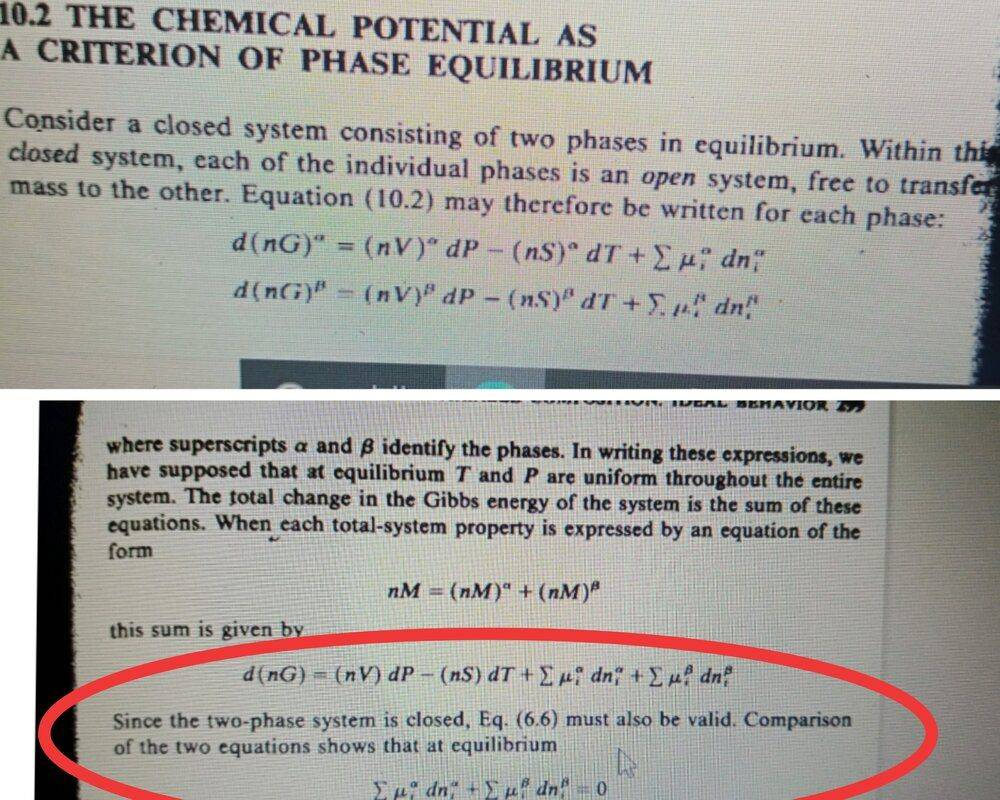In the first line of picture I uploaded it says consider a closed system consisting of two phase in equilibrium . That means we talking about two different phases where our species is being transferred from one to another, that is it isn't a system of homogeneous phase also we will not have a constant composition as at equilibrium composition might be different in the two phases . In the red curve I marked, it says cause its a closed system so we can use eq 6.6( the equation we talking about dg=vdp - sdT). But isn't this equation applicable only where there is homogeneous phase and constant composition ?

2) Let's say we have a closed system where water is boiling , I want to ask , the moment when the quality of steam has reached 0.5 , can we write dg(liq)=v(liq)dp- s(liq)dT+ μ(liq)dn(liq) , as of course during the process when the quality of steam reached from 0.1 to 0.5 , mass transfer has taken place, and the moles of liq water has reduced . But looking from other perspective this only liquid part in this system is homogeneous as well as constant composition as it is pure that is , this is applicable dg=vdp - sdT . I'm confused here , can't draw any further understanding out of it.

I have two doubts here on:

1)View attachment 267519

In the first line of picture I uploaded it says consider a closed system consisting of two phase in equilibrium . That means we talking about two different phases where our species is being transferred from one to another, that is it isn't a system of homogeneous phase also we will not have a constant composition as at equilibrium composition might be different in the two phases . In the red curve I marked, it says cause its a closed system so we can use eq 6.6( the equation we talking about dg=vdp - sdT). But isn't this equation applicable only where there is homogeneous phase and constant composition ?

Apparently, it also applies to any closed system (dn=0 for all species contained within the closed system).
2) Let's say we have a closed system where water is boiling , I want to ask , the moment when the quality of steam has reached 0.5 , can we write dg(liq)=v(liq)dp- s(liq)dT+ μ(liq)dn(liq) , as of course during the process when the quality of steam reached from 0.1 to 0.5 , mass transfer has taken place, and the moles of liq water has reduced . But looking from other perspective this only liquid part in this system is homogeneous as well as constant composition as it is pure that is , this is applicable dg=vdp - sdT . I'm confused here , can't draw any further understanding out of it.
In your first equation, is g the free energy per mole or the total free energy of n moles?

Lets consider it total free energy of n moles

Lets consider it total free energy of n moles
OK. Then write a similar equation for the vapor phase and add the two equations together. What do you get?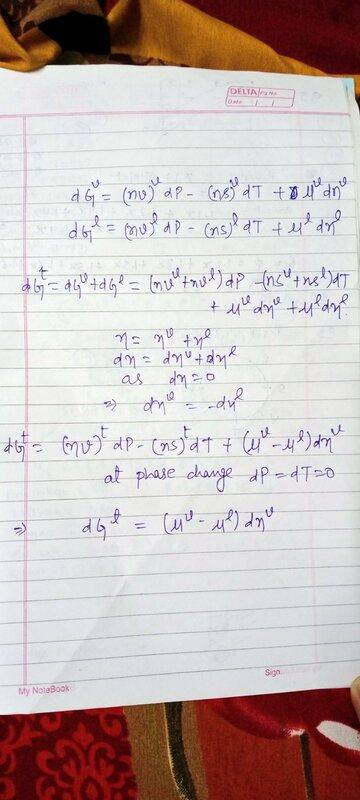Further in post#7 you proved that total gibbs free energy change is 0 during phase change , so further solving it will end up with μ(liq)=μ(vap).

View attachment 267527
Further in post#7 you proved that total gibbs free energy change is 0 during phase change , so further solving it will end up with μ(liq)=μ(vap).
Correct. And this equation also shows it because dnv is arbitrary.

So can we conclude that the only requirement to use this equation of Total Gibbs free energy change
d(ng)=(nv)dp - (ns)dT.
is that the system must be closed or if its open system then the Molar Gibbs free energy will remain constant provided the composition is constant .

So can we conclude that the only requirement to use this equation of Total Gibbs free energy change
d(ng)=(nv)dp - (ns)dT.
is that the system must be closed or if its open system then the Molar Gibbs free energy will remain constant provided the composition is constant .
If I understand you correctly, yes, in an open system, the molar Gibbs free energy will remain constant provided the composition is constant (at constant temperature and pressure). See the derivation of Eqns. 11.4 - 11.6 in your book.

I have a doubt from post#3 of this thread , you said homogeneous phase is the one where material is spatially uniform. Does that mean water and water vap mixture is a homogeneous phase? If not please give me one or two examples of homogeneous phase.

I have a doubt from post#3 of this thread , you said homogeneous phase is the one where material is spatially uniform. Does that mean water and water vap mixture is a homogeneous phase? If not please give me one or two examples of homogeneous phase.
Pure liquid water or pure water vapor are homogeneous phases. A homogeneous phase is spatially uniform (no variations in temperature, pressure, specific volume, composition)

Pure liquid water or pure water vapor are homogeneous phases. A homogeneous phase is spatially uniform (no variations in temperature, pressure, specific volume, composition)
That is we can say air is homogeneous phase right? And Sir what's is the difference between a substance of homogeneous phase with constant composition and Pure substance.

Last edited:
That is we can say air is homogeneous phase right? And Sir what's is the difference between a substance of homogeneous phase with constant composition and Pure substance.
The difference is that a substance of constant composition can be a solution, and not a material consisting of a single type of molecule.

But isn't it true that air is also a pure substance?

But isn't it true that air is also a pure substance?
No. Air is a mixture of several gases, mainly oxygen and nitrogen.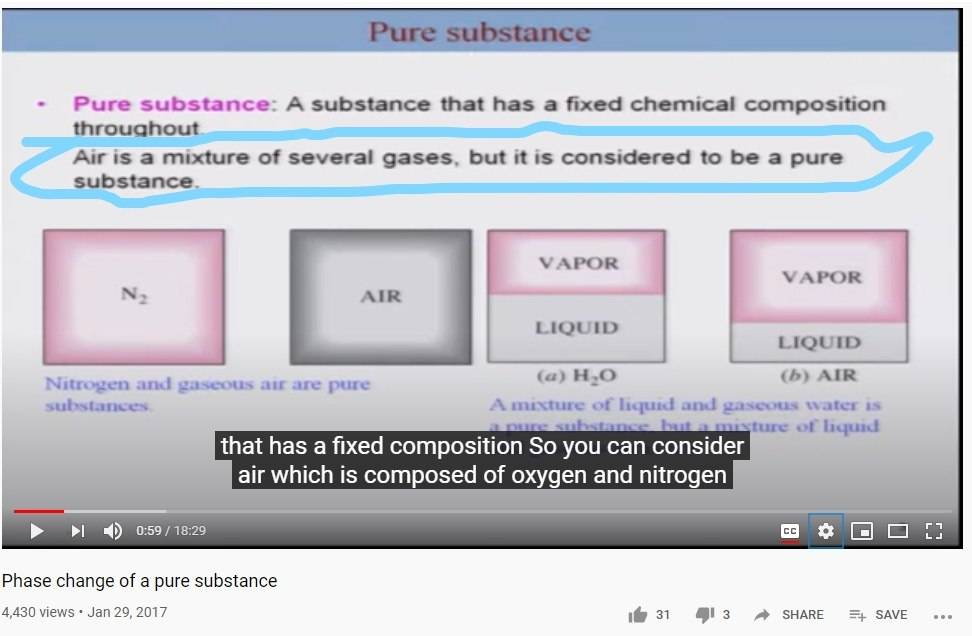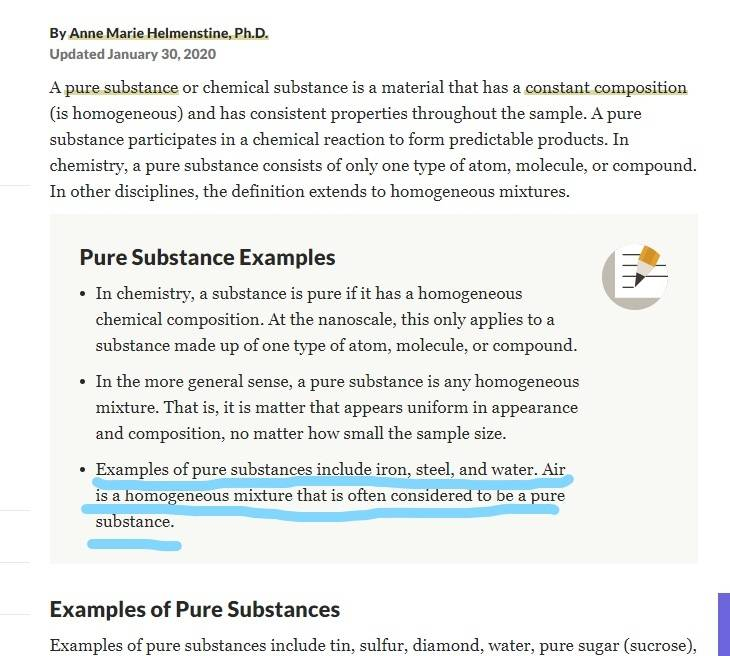I was actually super confused because of this above thing that I attached .

Can we conclude that
1)Air is a homogeneous phase with constant composition , liquid water or liq vapor separately taken are pure substances also separately they are homogeneous phase with constant composition while there liq water + water vap mixture is heterogeneous phase with constant composition ?

2) Pure substances are single component(either element or compound) in a homogeneous phase with constant composition .

3) Can I say a mixture of water and lemon juice( considering lemon juice as one component here for a while) mixed perfectly is an example of homogeneous phase with constant composition , while they both separately are pure substances ?

View attachment 267672
View attachment 267674
I was actually super confused because of this above thing that I attached .

Can we conclude that
1)Air is a homogeneous phase with constant composition , liquid water or liq vapor separately taken are pure substances also separately they are homogeneous phase with constant composition while there liq water + water vap mixture is heterogeneous phase with constant composition ?

2) Pure substances are single component(either element or compound) in a homogeneous phase with constant composition .

3) Can I say a mixture of water and lemon juice( considering lemon juice as one component here for a while) mixed perfectly is an example of homogeneous phase with constant composition , while they both separately are pure substances ?
These definitions don't conform to any thermodynamics book I've ever seen, and certainly not to Smith and van Ness, the textbook you were discussing earlier. So I leave it up to you to adopt whatever analysis and definitions please you.

These definitions don't conform to any thermodynamics book I've ever seen, and certainly not to Smith and van Ness, the textbook you were discussing earlier. So I leave it up to you to adopt whatever analysis and definitions please you.
Are my conclusions right? I'm not considering the definition from the pictures I attached , I'm following what you told me.

Can we conclude that
1)Air is a homogeneous phase with constant composition, liquid water or liq vapor separately taken are pure substances also separately they are homogeneous
phases
while liq water + water vap
is a heterogeneous mixture of pure phases.
2) Pure substances are single component(either element or compound) in a homogeneous phase with constant composition .
If they are pure, by definition they are of constant composition.
3) Can I say a mixture of water and lemon juice( considering lemon juice as one component here for a while) mixed perfectly is an example of homogeneous phase with constant composition , while they both separately are pure substances ?
yes, if you consider lemon juice as a single component. It is really as solution (mixture) of several pure organic substances (e.g., acetic acid)

Okay Sir Chet Got it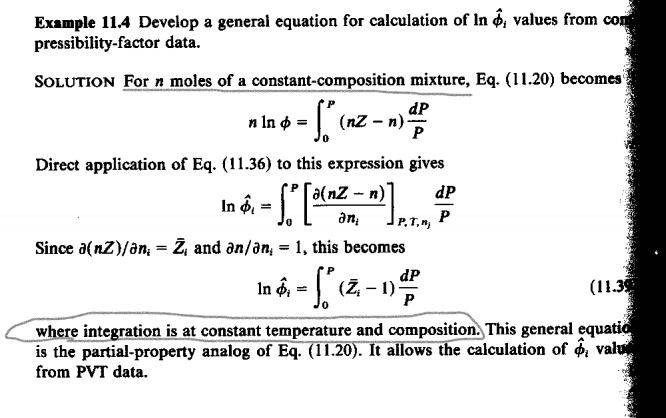Sir Chet here in this example it says ,For n moles of a constant composition mixture and integration is at constant composition. Does that mean we can't use this equation of fugacity coefficient for a system where there is change in composition occurring ?

Also, We know that Partial molar Gibbs energy for species i in solution is equal to RT times the
##ln\hat {\mathbf \phi_i } ## , and according to equation of partial molar property , partial molar property of a species i varies with the changes in composition of species i , hence ##ln\hat {\mathbf \phi_i } ## should also change with change in composition, But then this attached example talks about constant composition . I know I am missing something here, would you please help me out with this?

View attachment 267758

Sir Chet here in this example it says ,For n moles of a constant composition mixture and integration is at constant composition. Does that mean we can't use this equation of fugacity coefficient for a system where there is change in composition occurring ?
No. It means that this equation applies at any specified composition.
Also, We know that Partial molar Gibbs energy for species i in solution is equal to RT times the
##ln\hat {\mathbf \phi_i } ## , and according to equation of partial molar property , partial molar property of a species i varies with the changes in composition of species i , hence ##ln\hat {\mathbf \phi_i } ## should also change with change in composition, But then this attached example talks about constant composition . I know I am missing something here, would you please help me out with this?
As I said, this equation applies at any specific composition of the gas mixture. But, of course, it would give different values of the fugacity coefficient of a species for different mixture concentrations, temperatures, and pressures. Do you understand how it would be used in practice?

I would love to see an example , if you could tell me or just provide me a reference/link .

I would love to see an example , if you could tell me or just provide me a reference/link .
Suppose you have a mixture of gases, with specified mole fractions of these species, and you want to experimentally determine the fugacity coefficient for one of these species at the temperature and pressure of the mixture. Can you devise an experimental way of doing that using this equation?

I tried , but cannot think of any experimental way . I guess my domain of knowledge is restricted to theoretical part .

I tried , but cannot think of any experimental way . I guess my domain of knowledge is restricted to theoretical part .
What you would do is prepare 2 samples of the gas with n moles total in each. The number of moles of species i in each sample would then be ##n_{i}=nx_{i}##. In one of these samples you would then add a small amount of species i so ##n_{i}=nx_{i}+\delta##. You would then compress each of these samples at temperature T from low pressure to pressure P, and measure ##nZ_{i}=\frac{PV}{RT}## at each intermediate pressure along the way. Then, from the two samples at each pressure along the way, you would evaluate ##\bar{Z_i}=\frac{\Delta nZ_{i}}{\delta}## between the two samples. Then you would use these values to evaluate the integral.

•Rahulx084